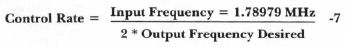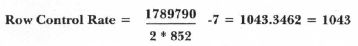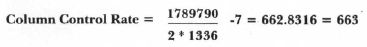`COMPUTE! ISSUE 30 / NOVEMBER 1982 / PAGE 150`

Computer Controlled Telephone Dialing

Mark Savarese
Livermore, CA

Use your Atari's sound generation capabilities to automatically dial a Touch Tone telephoneit's all done with software.

After playing a number of games on my Atari computer, I decided that writing a game with the quality of commercially available products might be pretty time consuming. So, I decided to do what I like best, tinkering.

I wanted a project that would be short but interesting and needed no hardware additions to my basic system. What uses computer-like sounds besides computer music? Why, telephone beeps, of course!

After a little research, I found that Touch Tone phone systems can be dialed by providing pairs of audio tones to the mouthpiece.

 1 2 3 4 Column # Frequency 1209 1336 1447 1663 Hz. 697 1 2 3 A 1 770 4 5 6 B 2 852 7 8 9 C 3 941 * 0 # D 4 Hz. Row #

Notice the fourth column (A,B,C,D). These buttons are not present on regular telephones, but tone pairs have been defined for them.

Suppose you wish to "dial" an 8. Just send two tones (at the same time) to the mouthpiece. An 8 would require one tone at 852 Hz and another tone at 1336 Hz.

Controlling Frequency

The Atari makes this a fairly simple task: simply send one tone on one voice and the other tone on a second voice. There is a complication, however. With normal tone generation, it is difficult to reproduce the exact frequencies needed. Never fear. The Atari provides a special mode to allow more precisely controlled frequency outputs. Two voices can be joined together to yield one "Double Precision Voice."

Line 370 connects the Atari's four voices and runs them as fast as possible to give a more precisely controlled output.

```340 REM CONNECT REGISTERS 1 AND 2 INTO ONE 16-BIT REGISTER.
350 REM CONNECT REGISTERS 3 AND 4 INTO ONE 16-BIT REGISTER.
360 REM CLOCK ALL FOUR REGISTERS AT 1.789790 MHZ (EXACTLY).
370 POKE 53768,120:REM SEE HARDWARE MANUAL.
```

The needed control rate can be calculated with the following formula:The control rate is used to produce a specific output frequency. The seven in the above formula is a fudge factor used when two sound registers are connected together.

In order to get the frequencies needed to dial an 8, calculate:Notice that the numbers were rounded to the nearest whole number. This rounding results in an error of less than 0.1 per cent.

Rather than have the Atari calculate two control rates for each digit to be dialed, I pre-calculated the rates needed for the eight possible frequencies and put these values into the array T.

While this technique requires a little more typing, it saves a significant amount of CPU time. (It is rather wasteful to recalculate the same eight values repeatedly. And an array of the eight frequencies would be required anyway.)

```170 DIM T(15,2):REM ETC.
```

The above dimension statement yields 16 sets of three values, which cover the 16 possible buttons on the telephone. Each phone button requires four bytes of information, but only three values need to be stored in the array because the fourth value is always a two by coincidence.

Here is an explanation of the program:

Lines

 0-140 Main program which calls two subroutines. 150-330 Load pre-calculated frequency control values into the array T. 340-400 Set up audio registers. 420-460 Get next "digit" to be dialed. 470-480 Convert "digit" to an AT ASCI I code number. 490-580 Convert "digit" to a number between 0 and 15 if it is legal, and call the tone output routine. 590 Return for a new user input if an illegal "digit" was entered. 600-700 Load frequency control values into the audio registers and output a tone pair.

These are the steps you take to use the program:

— Type the program in and SAVE it.

— Type RUN.

— The program will prompt you to enter a phone number.

— Type in the phone number you want (I suggest you use your own number to test).

— Make sure your TV volume is set moderately.

— Take your phone off the hook and check for the dial tone.

— Hold (or prop up) the mouthpiece very near your TV speaker.

— Finally, depress the return key on your computer.

— If nothing happens, you may need to adjust the TV volume up or move the phone closer to the TV speaker.

— Remember, only legal digits may be entered, no (,),- characters are allowed.

```0 REM PHONE DIALINB PROGRAM.
10 REM SET UP TONE TABLE AND SOUND RESISTERS.
20 GOSUB 150 30 REM ACCEPT AN INPUT FROM THE KEYBOARD.
4O PRINT
50 PRINT "ENTER PHONE NUMBER (UP TO 10 BUTTONS)"
60 PRINT "FROM O , 1 , 2 , 3 , 4 , 5 , 6, 7 , 8 , 9 , A , B, C, D, *,#"
70 PRINT "OR A CONTROL C TO EXIT THE PROGRAM.":PRINT
80 INPUT NO\$
90 REM CONVERT CHARACTER TO APPROPRIATE TONE NUMBER AND OUTPUT TONE.
100 GOSUB 430
110 REM IF LAST CHARACTER WAS NOT A C ONTROL C, GO BACK FOR THE NEXT CH ARACTER.
120 IF ASC(CH\$)<>3 THEN GOTO 40
130 SOUND 0,0,0,0:REM RESET SOUND.
140 END
150 REM SET UP THE TONE TABLE.
160 SOUND 0,0,0,0:REM INITIALIZE SOUN D REGISTERS,(REQUIRED).
170 DIM T(15,2):DIM CH\$(1):DIM NO\$(10)
18O T(0,0) = 151:T(0, l) = 3:T(0,2) = 176
190 T(1,0) = 221:T(1,1) = 4:T(1,2) = 253
200 T(2,0) = 151:T(2,1) = 4:T(2,2) = 253
210 T(3,0) = 87:T(3,1) = 4:T(3,2) = 253
220 T(4,0) = 221:T(4, 1) = 4:T(4,2) = 131
230 T(5,0) = 151:T(5, 1) = 4:T(5,2) = 131
240 T(6,0) = 87:T(6, 1) = 4:T(6,2) = 131
250 T(7,0) = 221:T(7.1) = 4:T(7,2) = 19
260 T(8,0) = 151:T(B,1) = 4:T(8,2) = 19
270 T(9,0) = 87:T(7,1) = 4:T(9,2) = 19
280 T(10,0) = 19:T(10,1) = 4:T(10,2) = 253
290 T(11,0) = 19:T(11,1) = 4:T(11,2) = 131
300 T(12,0) = 19:T(2,l) = 4:T(12,2) = 19
310 T(13,0) = 19:T(13,1) = 3:T(13,2) = 176
320 T(14,0) = 221:T(14.1) = 3:T(14,2) = 176
330 T(15,0) = 87:T(5,l) = 3:T(15,2) = 176
340 REM CONNECT REGISTERS 1 AND 2.
350 REM CONNECT REGISTERS 3 AND 4.
360 REM CLOCK ALL 4 REGISTERS AT 1.789790 MHZ.
370 POKE 53768,120
380 REM SET ALL VOLUMES TO ZERO.
390 POKE 53761,160:POKE 53763,160
400 POKE 53765,160:P0KE 53767,160
410 RETURN
420 REM CHECK FOR AN EMPTY STRING. 430 IF LEN(NO\$)<=O THEN RETURN
440 REM STRIP OFF LEFTMOST CHARACTER FROM THE STRING.
450 CH\$ = NO\$ ( 1 , 1 ) : IF LEN(NO\$)=1 THEN N0\$=" "
460 IF LEN(NO\$)<>0 THEN NO\$=NO\$(2)
470 REM CONVERT CHARACTER TO EQUIVALENT ATASCII CODE NUMBER.
480 CH=ASC(CH\$)
490 REM CONVERT TO A NUMBER BETWEEN 0 AND 15.
500 REM ADJUST IF 0 TO 9.
510 IF CH<=57 AND CH>=48 THEN TN=CH-48:GOSUB 61O:GOTO 430
520 REM ADJUST IF A TO D.
530 IF CH<=68 AND CH>=65 THEN TN=CH-55:GOSUB 61O:GOTO 430
540 REM ADJUST IF a TO d.
550 IF CH<=100 AND CH>=97 THEN TN=CH-87:GOSUB 610:GOTO 430
560 REM ADJUST IF # OR *.
570 IF CH\$="#" THEN TN=15:GOSUB 610:GOTO 430
580 IF CH\$="*" THEN TN=14:GOSUB 610:GOTO 43O
590 RETURN :REM RETURN IF ILLEGAL CHARACTER.
600 REM PUT TONE VALUES INTO SOUND REGISTERS.
610 POKE 53766,2:P0KE 53764,T(TN,0):POKE 53762,T(TN,1):POKE 5376O,T(TN,2)
620 REM TURN UP THE VOLUME ON REGISTRS 2 AND 4.
630 POKE 53767,168:POKE 53763,168
640 REM WAIT A SHORT TIME.
650 FOR 1=1 TO 5O:NEXT I
660 REM TURN OFF THE VOLUME ON REGISTERS 2 AND 4.
670 POKE 53767,160:POKE 53763,160
680 REM WAIT A SHORT TIME.
690 FOR 1=1 TO 1O:NEXT I
700 RETURN
```